### Example 10.7 Finding an Irreducible Infeasible Set

This example demonstrates the use of the IIS= option to locate an irreducible infeasible set. Suppose you want to solve a linear program that has the following simple formulation: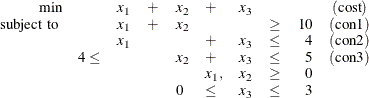The corresponding MPS-format SAS data set is as follows:


/* infeasible */
data exiis;
input field1 $field2$ field3 $field4 field5$ field6;
datalines;
NAME     .        .        .        .        .
ROWS     .        .        .        .        .
N       cost     .        .        .        .
G       con1     .        .        .        .
L       con2     .        .        .        .
G       con3     .        .        .        .
COLUMNS  .        .        .        .        .
.        x1       cost     1        con1     1
.        x1       con2     1        .        .
.        x2       cost     1        con1     1
.        x2       con3     1        .        .
.        x3       cost     1        con2     1
.        x3       con3     1        .        .
RHS      .        .        .        .        .
.        rhs      con1     10       con2     4
.        rhs      con3     4        .        .
RANGES   .        .        .        .        .
.        r1       con3     1        .        .
BOUNDS   .        .        .        .        .
UP       b1       x3       3        .        .
ENDATA   .        .        .        .        .
;


It is easy to verify that the following three constraints (or rows) and one variable (or column) bound form an IIS for this problem.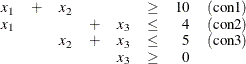You can use the IIS=ON option to detect this IIS by using the following statements:

proc optlp data=exiis
iis=on
primalout=iis_vars
dualout=iis_cons
logfreq=1;
run;


The OPTLP procedure outputs the detected IIS to the data sets specified by the PRIMALOUT= and DUALOUT= options, then stops. The notes shown in Output 10.7.1 are printed to the log.

Output 10.7.1: The IIS= Option: Log

 NOTE: The problem has 3 variables (0 free, 0 fixed). NOTE: The problem has 3 constraints (1 LE, 0 EQ, 1 GE, 1 range). NOTE: The problem has 6 constraint coefficients. NOTE: The IIS option is enabled. Objective                Entering      Leaving Phase Iteration        Value         Time     Variable      Variable P 1          1    1.400000E+01         0         x2           con3 (S) P 1          2    5.000000E+00         0         x1           con2 (S) P 1          3    1.000000E+00         0 P 1          4    1.000000E+00         0 NOTE: The IIS option found the problem to be infeasible. NOTE: Applying the IIS sensitivity filter. NOTE: The sensitivity filter removed 1 constraints and 3 variable bounds. NOTE: Applying the IIS deletion filter. NOTE: Processing constraints. Processed     Removed      Time 0           0         0 1           0         0 2           0         0 3           0         0 NOTE: Processing variable bounds. Processed     Removed      Time 0           0         0 1           0         0 2           0         0 3           0         0 NOTE: The deletion filter removed 0 constraints and 0 variable bounds. NOTE: The IIS option found the problem to be infeasible. NOTE: The IIS option found an irreducible infeasible set with 1 variables and 3 constraints. NOTE: The IIS solve time is 0.03 seconds. NOTE: The data set WORK.IIS_VARS has 3 observations and 10 variables. NOTE: The data set WORK.IIS_CONS has 3 observations and 10 variables.

The data sets iis_cons and iis_vars are shown in Output 10.7.2.

Output 10.7.2: Identify Rows and Columns in the IIS

 Constraints in the IIS

Obs Objective
Function ID
RHS ID Constraint
Name
Constraint
Type
Constraint
RHS
Constraint
Lower
Bound
Constraint
Upper
Bound
Dual Variable
Value
Constraint
Status
Constraint
Activity
1 cost rhs con1 G 10 . . . I_L .
2 cost rhs con2 L 4 . . . I_U .
3 cost rhs con3 R . 4 5 . I_U .

 Variables in the IIS

Obs Objective
Function ID
RHS ID Variable
Name
Variable
Type
Objective
Coefficient
Lower
Bound
Upper Bound Variable
Value
Variable
Status
Reduced
Cost
1 cost rhs x1 N 1 0 1.7977E308 .   .
2 cost rhs x2 N 1 0 1.7977E308 .   .
3 cost rhs x3 D 1 0 3 . I_L .

The constraint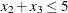, which is an element of the IIS, is created by the RANGES section. The original constraint is con3, a
constraint with an RHS value of 4. If you choose to remove the constraint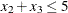, you can accomplish this by removing con3 from the RANGES section in the MPS-format SAS data set exiis. Since con3 is the only observation in the section, the identifier observation can also be removed. The modified LP problem is specified in the following SAS statements:

 /* dropping con3, feasible */
data exiisf;
input field1 $field2$ field3 $field4 field5$ field6;
datalines;
NAME     .        .        .        .        .
ROWS     .        .        .        .        .
N       cost     .        .        .        .
G       con1     .        .        .        .
L       con2     .        .        .        .
G       con3     .        .        .        .
COLUMNS  .        .        .        .        .
.        x1       cost     1        con1     1
.        x1       con2     1        .        .
.        x2       cost     1        con1     1
.        x2       con3     1        .        .
.        x3       cost     1        con2     1
.        x3       con3     1        .        .
RHS      .        .        .        .        .
.        rhs      con1     10       con2     4
.        rhs      con3     4        .        .
BOUNDS   .        .        .        .        .
UP       b1       x3       3        .        .
ENDATA   .        .        .        .        .
;


Since one element of the IIS has been removed, the modified LP problem should no longer contain the infeasible set. Due to the size of this problem, there should be no additional irreducible infeasible sets. You can confirm this by submitting the following SAS statements:

proc optlp data=exiisf
pout=po
iis=on;
run;


The notes shown in Output 10.7.3 are printed to the log.

Output 10.7.3: The IIS= Option: Log

 NOTE: The problem has 3 variables (0 free, 0 fixed). NOTE: The problem has 3 constraints (1 LE, 0 EQ, 2 GE, 0 range). NOTE: The problem has 6 constraint coefficients. NOTE: The IIS option is enabled. Objective Phase Iteration        Value         Time P 1          1    1.400000E+01         0 P 1          3    0.000000E+00         0 NOTE: The IIS option found the problem to be feasible. NOTE: The IIS solve time is 0.05 seconds. NOTE: The data set WORK.EXSS has 8 observations and 3 variables. NOTE: The data set WORK.PO has 3 observations and 10 variables.

The solution summary and the primal solution are displayed in Output 10.7.4.

Output 10.7.4: Infeasibility Removed

 Solution Summary

Obs Label1 cValue1 nValue1
1 Solver LP .
2 Algorithm Primal Simplex .
3 Objective Function cost .
4 Solution Status Feasible .
5     .
6 Iterations 3 3.000000
7 Presolve Time 0.00 0
8 Solution Time 0.05 0.046800

 Primal Solution

Obs Objective
Function ID
RHS ID Variable
Name
Variable
Type
Objective
Coefficient
Lower
Bound
Upper Bound Variable
Value
Variable
Status
Reduced
Cost
1 cost rhs x1 N 1 0 1.7977E308 .   .
2 cost rhs x2 N 1 0 1.7977E308 .   .
3 cost rhs x3 D 1 0 3 .   .